# Model Evaluation for Classification in Machine Learning

## Model Evaluation for Classification in Machine Learning

In this article, I am going to discuss Model Evaluation for Classification in Machine Learning with Examples. Please read our previous article where we discussed the Decision Tree in Machine Learning with Examples.

##### Model Evaluation for Classification in Machine Learning
###### Accuracy –

In classification problems, accuracy refers to the number of correct predictions made by the model across all types of predictions.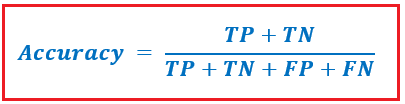The numerator contains our correct predictions (True positives and True negatives) (shown in red in the diagram above), while the denominator contains all of the algorithm’s forecasts (Right as well as wrong ones).

###### When to Use Accuracy?

When the target variable classes in the data are approximately balanced, accuracy is a good measure. For example, apples account for 60% of our fruit image data, while oranges account for 40%.

###### When to Avoid Accuracy?

When the target variable classes in the data are a majority of one class, accuracy should never be utilized as a measure.

In our cancer detection scenario, only 5 persons out of 100 have cancer. Let’s pretend our model is terrible and every instance is predicted to be cancer-free. As a result, it properly identified 95 non-cancerous patients and 5 cancerous patients as non-cancerous. Even though the model is horrible at predicting cancer, it has a 95% accuracy rate.

##### Precision –

Precision is a metric that indicates what percentage of patients diagnosed with cancer actually have cancer. People who are expected to be malignant (TP and FP) and those who actually have cancer are both TP.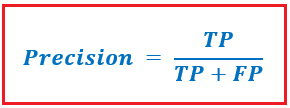In our cancer scenario, only 5 persons out of 100 have cancer. Let’s pretend our model is terrible and every instance is diagnosed with cancer. Our denominator (True positives and False Positives) is 100, while the numerator (individual with cancer and model predicting his case as cancer) is 5. In this case, we can say that the precision of the model is 5%.

If we want to focus on reducing False Negatives, we’ll want our Recall to be as near to 100 percent as feasible without sacrificing precision.

##### Recall –

The Recall is a metric that indicates how many patients with cancer were mistakenly diagnosed as having cancer by the algorithm. Actual positives (people with cancer are TP and FN) and persons diagnosed with cancer by the model are both TP. (Note: FN is included because the Person was diagnosed with cancer against the model’s prediction.)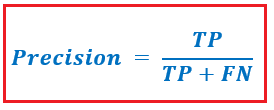Example: Of the 100 people in our cancer example, only 5 have cancer. Let’s imagine the model predicts cancer in every case. If we want to reduce False Positives, we’ll want Precision to be as close to 100 percent as possible.

##### F1 Score –

When we create a model to solve a classification problem, we don’t want to carry both Precision and Recall in our wallets. So, if we can get a single score that represents both Precision(P) and Recall(R), that would be ideal (R). Taking their arithmetic mean is one approach to do this. (P + R) / 2, where P stands for Precision and R stands for Recall. However, in some circumstances, this is not a good thing.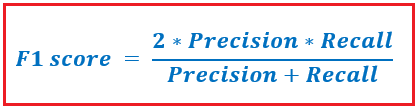Let’s imagine we have 100 credit card transactions, 97 of which are legitimate and 3 of which are fraudulent, and we developed a model that forecasts all of them as fraud.

If one of the numbers between precision and recall is extremely little, the F1 Score raises a red flag and is more similar to the smaller number than the larger one, providing the model a suitable score rather than just an arithmetic mean.

##### AUC-ROC Curve –

Assume we have 100 credit card transactions, 97 of which are valid and 3 of which are fraudulent, and we have constructed a model that predicts all of them to be fraudulent.

The F1 Score raises a red flag if one of the precision and recall numbers is extremely small, and it is more comparable to the smaller number than the bigger one, giving the model a proper score rather than merely an arithmetic mean.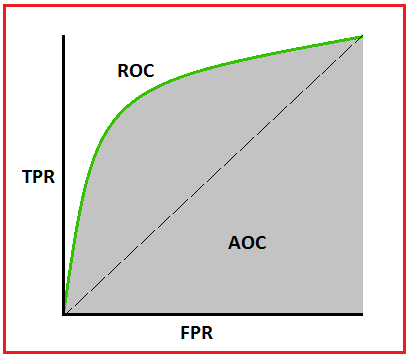An excellent model has an AUC close to 1, indicating that it has a high level of separability. AUC approaching 0 indicates a bad model, which has the lowest measure of separability. It predicts 0s to be 1s and 1s to be 0s. When AUC = 0.5, the model has no ability to distinguish between classes.

Let’s put the following statements into context. ROC is a probability curve, as we all know. So, here’s how those probabilities’ distributions look:

The positive class (people with the disease) has a red distribution curve, while the negative class has a green distribution curve (patients with no disease).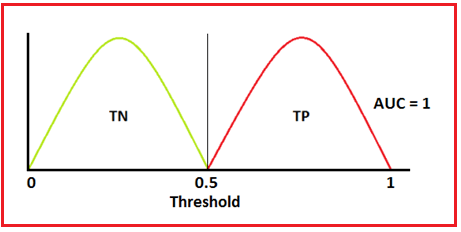This is a perfect scenario. The model has an optimum measure of separability when two curves do not overlap at all. It can tell the difference between positive and negative classes with ease.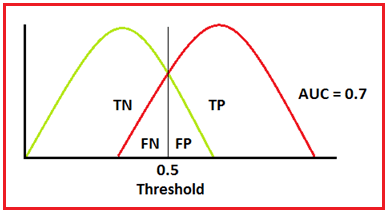We introduce type 1 and type 2 mistakes when two distributions overlap. We can minimize or maximize them depending on the threshold. When the AUC is 0.7, the model has a 70% chance of distinguishing between positive and negative classes.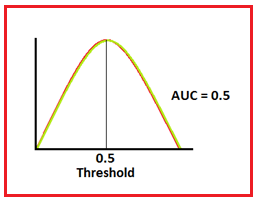This is the worst-case scenario. The model has no discrimination capacity to distinguish between positive and negative classes when AUC is around 0.5.

In the next article, I am going to discuss Random Forests in Machine Learning with Examples. Here, in this article, I try to explain Model Evaluation for Classification in Machine Learning with Examples. I hope you enjoy this Model Evaluation for Classification in Machine Learning with Examples article.